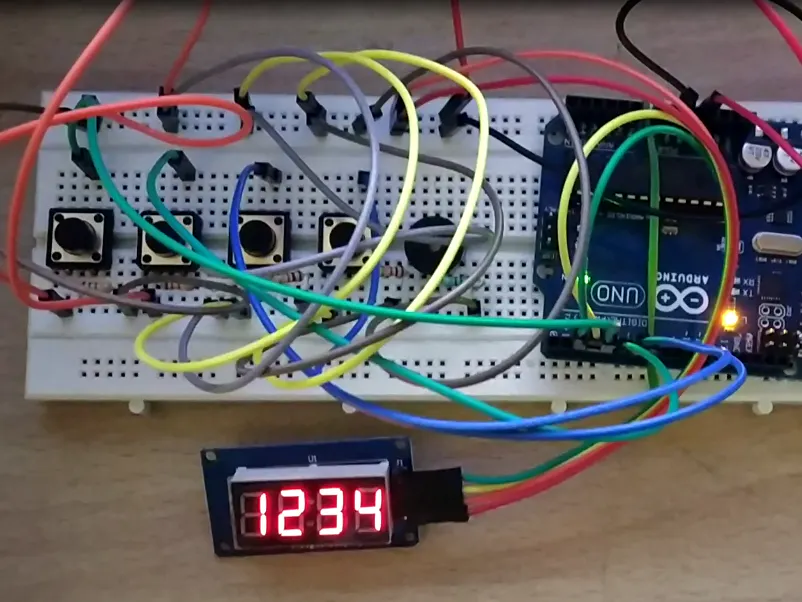Project showcase# Turning Lock Puzzle

Unlock a 4-digit dial with known password 0000.

• 3,413 views
• 4 respects

## Components and supplies

Turning Lock is small puzzle inspired by generic 4-digit dial used to lock luggage bags. Usually, other than the person who set the password before locking, no one else can crack the password, unless uses exhaustive permutations 10x10x10x10. Surprisingly this lock has the password always set to 0000, twist is that turning each of individual digit or dial will turn not only the self but also its left/right neighbours. Note that each dial is circular with numbers 0, 1, 2, 3, 4, 5, 6, 7, 8, 9.

The objective of this puzzle is set the dials to 0000 from a random state at the beginning when reset. Each dial is circular and connected to adjacent neighbours. Increment a dial by one will also increment the connected adjacent neighbours by one.

Arduino is connected to TM1637 (contains 4 digit 7 segment led display). Each button represent each digit. Button press lead to increment of associated digit in circular fashion by one. Turning all four digits to 0000 unlocks the puzzle with LED glow.

## Code

##### Turning_Lock_with_TM1637Arduino
```#include <Arduino.h>
#include <TM1637Display.h>

#define CLK 2
#define DIO 3

TM1637Display display(CLK, DIO);

void setup()
{
pinMode(4, INPUT); // Button-1 for Digit in 1000 Place
pinMode(5, INPUT); // Button-1 for Digit in 100 Place
pinMode(6, INPUT); // Button-1 for Digit in 10 Place
pinMode(7, INPUT); // Button-1 for Digit in 1 Place
pinMode(9, OUTPUT);

display.setBrightness(2);
Serial.begin(9600);
}

long numb = 1234; //random(1000,9999);

int pres4 = 0;
int pres5 = 0;
int pres6 = 0;
int pres7 = 0;

int numb4 = 0;
int numb5 = 0;
int numb6 = 0;
int numb7 = 0;

void loop()
{
display.showNumberDec(numb, true);
delay(100);

//breaking 4-digited number into individual digits
numb4 = numb / 1000;
numb5 = (numb / 100) % 10;
numb6 = (numb / 10) % 10;
numb7 = numb % 10;

//When Button is pressed, there is an Increment in Digits (Self + Adjacent Neighbors).
//Digits represent Dial 0,1,2,3,4,5,6,7,8,9,0 etc.
//Special Case: Incrementing 9 by 1 leads to 10, therefore need to reduce the left neighbor by 1.

if (pres4 == 1) {
numb = numb + 1100;	//Digit in 1000 place increment by 1
if (numb5 == 9) {
numb = numb - 1000;	//Digit in 100 place decrement by 1
}
pres4 = 0;
}

if (pres5 == 1) {
numb = numb + 1110;	//Digit in 100 place increment by 1
pres5 = 0;
if (numb5 == 9) {
numb = numb - 1000;	//Digit in 100 place decrement by 1
}
if (numb6 == 9) {
numb = numb - 100;	//Digit in 10 place decrement by 1
}
}

if (pres6 == 1) {
numb = numb + 111;	//Digit in 10 place increment by 1
pres6 = 0;
if (numb6 == 9) {
numb = numb - 100;	//Digit in 10 place decrement by 1
}
if (numb5 == 9) {
numb = numb - 1000;	//Digit in 1000 place decrement by 1
}
if (numb7 == 9) {
numb = numb - 10;	//Digit in 1 place decrement by 1
}
}

if (pres7 == 1) {
numb = numb + 11;	//Digit in 1 place increment by 1
pres7 = 0;
if (numb7 == 9) {
numb = numb - 10;	//Digit in 1 place decrement by 1
}
if (numb6 == 9) {
numb = numb - 100;	//Digit in 10 place decrement by 1
}
}

if (numb > 9999) {
numb = numb - 10000 * (numb / 10000);
}

Serial.println(numb);
//LED to Glow or Buzzer to Ring
if (numb == 0) {
digitalWrite(9, HIGH);
}
else {
digitalWrite(9, LOW);
}
}
```

## Schematics

turning_lock_with_tm1637_FdbE0lV52I.fzz

#### Published on

June 5, 2019
See similar projects
you might like

#### Grid Lock Puzzle

Project showcase by Krishna Lalith

• 1,746 views
• 3 respects

#### Infrared Controlled Logic Puzzle -- Lights On!

Project tutorial by FIELDING

• 4,782 views
• 15 respects

#### RFID Desk Lamp with RGB Color Scheme Lock

Project tutorial by Kutluhan Aktar and JLCPCB

• 2,886 views
• 1 comment
• 5 respects

#### Door Lock System with Arduino

Project showcase by jayesh_nawani

• 58,133 views
• 32 respects

#### LED Dice

Project showcase by EvdS

• 63,841 views